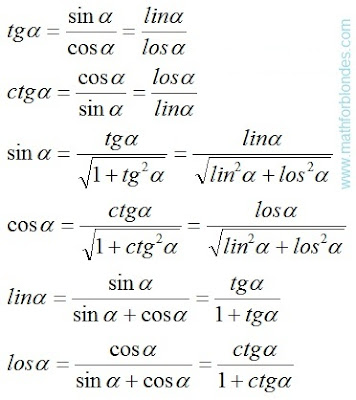## 8.03.2016

### Three main types of trigonometric functions

Subject of occupations:
TRIGONOMETRIC FUNCTIONS IN A RECTANGLE
Subject of the previous lesson
Angular symmetry in a rectangle

Lesson 3

Three main types of trigonometric functions

Trigonometric functions are the dependences between angles and numbers in a rectangle expressed in own units of measure. Own unit of measure is one of characteristics of object, accepted as a unit of measure.

In a rectangle it is possible to allocate three main types of trigonometric functions:

- the infinite trigonometric functions – a tangent and a cotangent, geometrically it:

a) the size of one of the parties of a rectangle at simple value of length of other party, is reflected by communication of numbers and units of measure;

b) the sizes of the parties of a rectangle at simple area, reflect laws for multiplication;

- terminating trigonometric functions – the sine and a cosine, geometrically are the sizes of the parties of a rectangle at simple diagonal, reflect projective properties of space;

- the linear angular functions – the linear sine (lin) and the linear cosine (los), geometrically is the sizes of the parties of a rectangle at a simple semiperimeter, reflect laws of addition.

Other types of trigonometric functions in this work are not considered as they did not draw attention of the author. All trigonometric functions establish particular connection between angles and numbers. It is quite possible that the mathematics of some extraterrestrial civilizations can be constructed on angles in the same way as at us it is constructed on numbers.

Expression of the trial trigonometric functions through the parties of a rectangle.Expression of the trial trigonometric functions through the parties of a rectangle

The main ratios on types of trigonometric functions.The main ratios on types of trigonometric functions

Values of trigonometric functions of some angles.Values of trigonometric functions of some angles

Expression of one trigonometric functions through others.Expression of one trigonometric functions through others

The explanation for visitors of this website. If to look at formulas of a tangent and a cotangent (the last picture), then can seem that between a sine with a cosine and linos with loses there is no difference. But you do not hurry with conclusions. If two fractions are equal, it does not mean at all that their numerators and denominators are equal. Let's review an example. Two thirds are equal to four sixth. But two it is not equal to four, three it is not equal to six.

In more detail the trial trigonometric functions are considered below.

At the following lesson we will consider
Terminating trigonometric functions